# Study on the wetting effect of the silty clay reservoir bank sub-grade

## Dahe Qi1, Xinjian Yang2

1Chongqing Metropolitan College of Science and Technology, ChongQing, China

2Water Administration Section of Yangping Irrigation Area Management, ChangDe, China

1Corresponding author

Vibroengineering PROCEDIA, Vol. 44, 2022, p. 93-98. https://doi.org/10.21595/vp.2022.22722
Received 22 May 2022; received in revised form 21 June 2022; accepted 27 June 2022; published 25 August 2022

58th International Conference on Vibroengineering, Ventspils, Latvia, August 25-26, 2022

Copyright © 2022 Dahe Qi, et al. This is an open access article distributed under the Creative Commons Attribution License, which permits unrestricted use, distribution, and reproduction in any medium, provided the original work is properly cited.
Views 6
CrossRef Citations 0
Abstract.

In this paper, the deformation characteristics of silty clay after immersion in water were studied. The wetting test of silty clay in a stratum in Chongqing under different confining pressures and different stress levels was carried out by using multi functional triaxial apparatus. Through the single line method and double line method experiments, the law of wetting deformation of silty clay was obtained and a new curve formula was fitted.

Highlights
• The single-line method can truly reflect the wetting process of silty clay.
• The relationship between axial strain and stress level can be expressed by exponential function.
• The correlation of test parameters is good.

Keywords: silty clay, wetting deformation, single-line method.

#### 1. Introduction

Silty clay is widely distributed in many areas in many countries. Silty clay also widely exists on the banks of the Yangtze River and Jialing River in Chongqing. The sub-grade of reservoir bank highway is infiltrated by river water all year round. The physical and mechanical properties of silty clay often affect the stability and reliability of soil mass. Under the influence of long-term rainfall infiltration or the rise of groundwater level, the silty clay mass is soaked and wetted, lead to wetting deformation.

Wetting deformation  refers to the rearrangement and position adjustment of soil particles under the action of water immersion, resulting in additional deformation and additional settlement of embankment, lead to deformation and cracking of pavement, and even instability and failure of embankment slope. Therefore, the study on the wetting characteristics of silty clay is of great engineering significance to silty clay reservoir bank sub-grade. Nobari and Duncan  proposed using dry and wet stress-strain curves to calculate wetting deformation through experiments. Z. Z. Yin  proposed an improved humidification calculation model on this basis. The wetting deformation characteristics of soil are related to the type of soil, wetting path and stress path. At present, the research on wetting deformation is mainly focused on coarse-grained materials  and rock-fill materials . X. J. Chen et al.  studied the effect of water content and dry density on strength characteristics of silty clay. X. L. Man et al.  studied the effect of silt content in filling sand on the geotubes dewatering performance, and X. C. Zhang et al.  studied the wetting deformation of cohesive soil of an expressway sub-grade under different stress paths. X. J. Chen studied the influence of water content and dry density on the strength characteristics of silty clay .

At present, there is little research on the wetting deformation of silty clay. In this paper, according to the sub-grade of silty clay reservoir bank, the tests of single line method and double line method are carried out, and the results were analyzed and studied.

#### 2. Triaxial wetting deformation test

The confining pressure of the test is 100 kPa, 200 kPa, 300 kPa. The undisturbed soil sample is fixed on the test bench, and then the confining pressure is applied to conduct triaxial drainage shear test on the sample. When the total axial stress reaches the total stress of the corresponding unsaturated consolidation drainage shear failure, That is, when the stress level s is 0.2, 0.35, 0.5 and 0.75, stop the axial shear force and keep the surrounding pressure and total axial stress unchanged, when the axial strain is less than 0.1 % per hour, the sample begins to saturate from bottom to top, and the soaking process is the wetting process, in this process, the confining pressure and total axial stress remain unchanged. The wetting deformation is the deformation at the end of wetting minus the deformation at the beginning of wetting. Then continue the triaxial drained shear test on the sample until the sample is damaged. See Fig. 1 for the wetting loading scheme.

As for the process and method of water saturation, the comparative test of head saturation method and back pressure saturation method was carried out in the early stage , Therefore, the back pressure saturation method is used to saturate the soil sample in the process of triaxial wetting test. The sample used in the test is the Quaternary Holocene alluvium silty clay in the riverbed of Jialing River. The basic physical property indexes are shown in Table 1, and the state of the sample before and after the test is shown in Fig. 1.

Table 1. Basic physical property of soil samples

 Density Moisture content Dry density Proportion g/cm3 % g/cm3 1.82 20.10 1.52 2.734 Liquid limit Plastic limit Saturated moisture Void ratio % % % 38.74 19.16 29.36 0.803

#### 3. Analysis of experimental results of single-line and double-line method

According to the author's experimental design, the tests of single-line method and double-line method are carried out respectively. The confining pressure of single-line method test is 100 kPa, 200 kPa and 300 kPa, Stress level $S=$ 0.2, 0.35, 0.5, 0.75. The confining pressure of double-line method is the same.

Fig. 1. The test piece before and after axial wetting test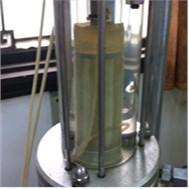a) Before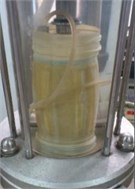b) After

#### 3.1. Test results of single-line method

As the test instrument is a strain controlled triaxial apparatus, it is impossible to finely reach the deviatoric stress value of the design stress level when controlling the axial loading, and the actual stress level shall prevail in the subsequent analysis. Fig. 2 shows the s ingle line method curve under different confining pressures, and Fig. 3 shows the volume curve.

The test results show that the basic law of wetting deformation is as follows: The stress-strain curve in the wetting stage is similar to the horizontal line, under the same confining pressure, the axial strain and volume deformation increase with the increase of stress level. The wetting volume deformation shows shear shrinkage, but the volume deformation increases approximately linearly in the wetting stage. Due to the low confining pressure of the test, the maximum is 300 kPa, so the whole process stress-strain curve of silty clay is hardening type.

After wetting, in the shear stage, the stress-strain curve of soil sample first increases linearly, and then shows nonlinear characteristics until failure. In the process of wetting, the deformation of soil belongs to plastic deformation, and the stress state of soil after wetting has not changed, but its yield surface within the plastic range has expanded and a new yield surface has been generated. Therefore, during the shear loading stage, the soil is elastically deformed to a new yield surface before plastic deformation.

Fig. 2. Single-line method curve under different confining pressures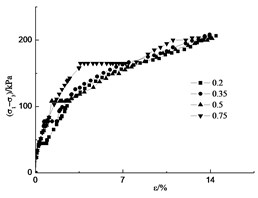a) Confining pressure 100 kPa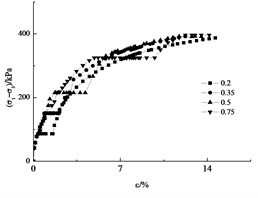b) Confining pressure 200 kPa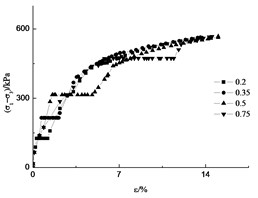b) Confining pressure 300 kPa

Fig. 3. Single-line method volume change curve under different confining pressures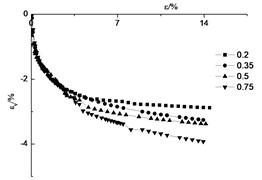a) Confining pressure 100 kPa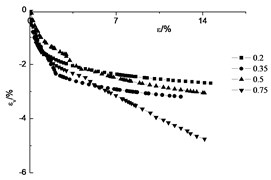b) Confining pressure 200 kPa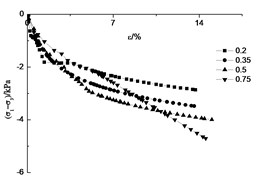b) Confining pressure 300 kPa

#### 3.2. Test results of double-line method

In the double line method, three confining pressure was applied 100 kPa, 200 kPa and 300 kPa, and the dry and wet samples were applied respectively.

Fig. 4. Double-line method volume change curve under different confining pressures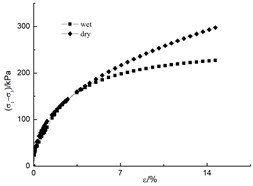a) Confining pressure 100 kPa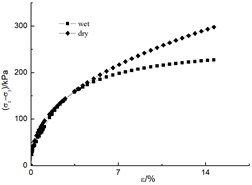b) Confining pressure 200 kPa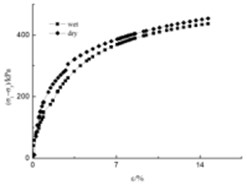b) Confining pressure 300 kPa

Fig. 5. Double-line method volume deformation under different confining pressures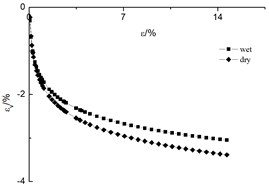a) Confining pressure 100 kPa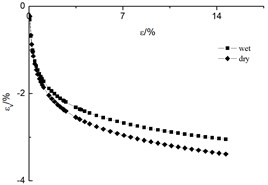b) Confining pressure 200 kPa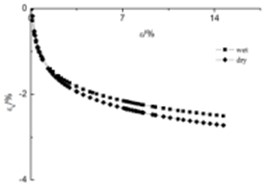b) Confining pressure 300 kPa

The test results show the stress-strain curve law of axial deformation and volume deformation is the same as that of single-line method, as shown in Fig. 4 and Fig. 5.

#### 4. Comparison between single-line method and double-line method

In essence, the double-line method is a shear test of two samples with different initial moisture content. It does not consider the wetting deformation caused by the process of moisture wetting, but also changes the action sequence of water and load on the soil sample, which is inconsistent with the actual stress state and soaking process of silty clay. Most of the deformation in the actual project is produced in the process of the interaction between load and wetting.

Fig. 6 shows that the test results of the double-line method are small, it is unsafe to use it for design. The single-line method is a test based on the stress state of the soil when it is actually immersed in water. The test results are closer to the actual deformation of the soil, which should be more reasonable than the double-line method. But the single line method needs to carry out many groups of tests through different confining pressures and different stress levels to obtain the wetting deformation, which is a little deficiency.

Fig. 6. Comparison between single-line method and double-line method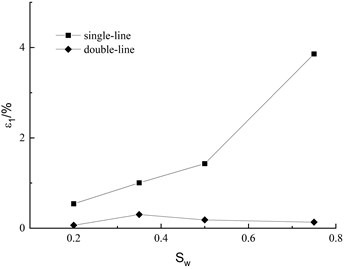a) Axial deformation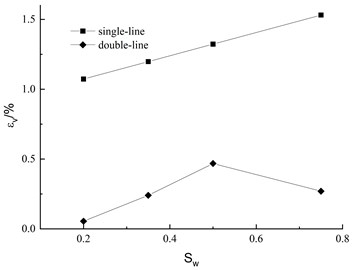b) Volumetric deformation

Chinese scholar Shen Zhujiang once suggested a wetting deformation model , which is as follows:

(1)
$∆{\epsilon }_{v}=C,$
(2)
$∆{\epsilon }_{s}={d}_{w}\frac{S}{1-S},$
(3)
$S=\frac{{\sigma }_{1}-{\sigma }_{3}}{{\left({\sigma }_{1}-{\sigma }_{3}\right)}_{t}},$

where $S$ is the stress level of immersion test, $C$, ${d}_{w}$ are two coefficients, and it is suggested that the volumetric strain coefficient be measured by the compression instrument. Eq. (1) shows that the wetting volume strain $∆{\epsilon }_{v}$ is a constant, independent of the stress state

The volume deformation test data of silty clay in this paper show that $∆{\epsilon }_{v}$ varies not only with stress level, but also with confining pressure.

Eq. (2) shows that the axial shear strain of wetting is only related to the stress level, which is a hyperbolic relationship. The test data in this paper show that the axial strain and stress level of wetting of silty clay are exponential functions, and Shen Zhujiang model cannot well describe the wetting deformation of silty clay.

The relationship between additional axial deformation and stress level caused by wetting of silty clay is shown in Fig. 6, which can be expressed by the following formula:

(4)
$∆{\epsilon }_{s}^{w}=∆{\epsilon }_{w}-∆{\epsilon }_{s}=H\mathrm{*}{e}^{{S}_{w}m},$

where parameter $H$, $m$ is determined by test.

Fig. 7. Additional deformation caused by wetting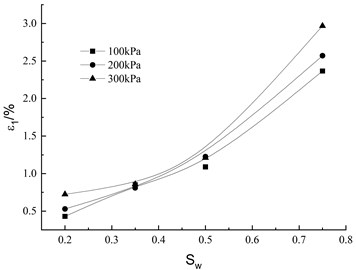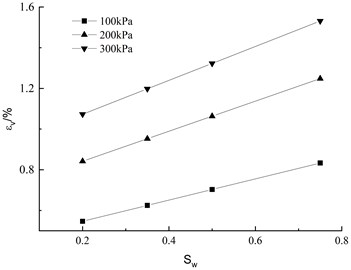b) Volumetric deformation

From Fig. 7, we can get the volume deformation fitting formula:

(5)
$∆{\epsilon }_{v}^{w}=C{S}_{w}+D,$

where: $C$ and $D$ are test parameters related to confining pressure, which can be expressed as:

(6)
$C=c\left(\frac{{\sigma }_{3}}{{P}_{a}}\right)+d,$
(7)
$D=f\left(\frac{{\sigma }_{3}}{{P}_{a}}\right)+g,$

where $c$, $d$, $f$, $g$ is constant determined by test.

Fig. 8. Parameters C and D with ${\sigma }_{3}/{P}_{a}$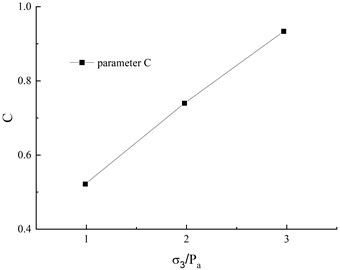a) Parameters C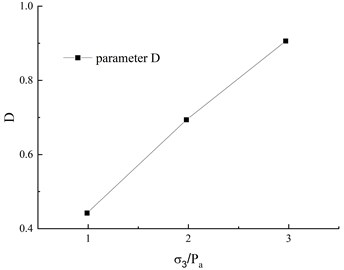b) Parameters D

#### 5. Conclusions

Based on the single line method and double line method, the water immersion deformation test of silty clay in Chongqing is carried out. The results show that:

1) The double-line method cannot reflect the actual soaking process of soil samples, and the test results are small, which is not conducive to the optimal design. The single-line method can truly reflect the soaking process of soil, which is better than the double line method.

2) Shen Zhujiang model is not suitable for silty clay. A new fitting formula is proposed to describe the additional axial deformation caused by wetting of silty clay and the volumetric deformation under different wetting stress levels. The test parameters related to confining pressure have good correlation.

Further experimental research will be carried out in the follow-up, and whether there is creep deformation in the wetting deformation will be considered.

1. G. X. Li, “Slacking test and math model of rock fill,” (in Chinese), Chinese Journal of Geo-technical Engineering, Vol. 12, No. 5, pp. 58–64, 1990. [Search CrossRef]
2. E. S. Nobari and J. M. Duncan, “Movements in dams due to reservoir filling,” in Performance of Earth and Earth-Supported Structures, p. 797, 1973. [Search CrossRef]
3. Z. Z. Yin and H. Zhao, “Deformation analysis of earth dam during reservoir filling,” (in Chinese), Chinese Journal of Geo-technical Engineering, Vol. 12, No. 2, pp. 1–8, 1990. [Search CrossRef]
4. P. Li, Z. Li, and J. Y. Liu, “Slaking test study of coarse aggregate under high triaxial stress condition,” (in Chinese), Chinese Journal of Rock Mechanics and Engineering, Vol. 23, No. 2, pp. 231–234, 2004. [Search CrossRef]
5. H. Fu, H. Q. Han, and X. J. Zhao, “Experimental study on slaking deformation properties of rock-fill,” (in Chinese), Journal of Water Resources and Architectural Engineering, Vol. 8, No. 4, pp. 50–53, 2010. [Search CrossRef]
6. J. M. Chang and R. Y. Yin, “Study on backbone curve of silty clay in heavy-haul railway subgrade considering wetting,” (in Chinese), Journal of The China Railway Society, Vol. 42, No. 7, pp. 141–147, 2020. [Search CrossRef]
7. X. Man, S. Hu, and W. Wang, “Effect of silt content in filling sand on the geotubes dewatering performance by hanging bag tests,” (in Chinese), Journal of Measurements in Engineering, Vol. 8, No. 3, pp. 132–141, Sep. 2020, https://doi.org/10.21595/jme.2020.21645 [Publisher]
8. X. C. Zhang, Y. Z. Wang, and X. D. Fu, “Experimental research on wetting deformation of clayey soil of a freeway sub-grade with different stress paths,” (in Chinese), Rock and Soil Mechanics, Vol. 31, No. 6, pp. 1791–1796, 2010. [Search CrossRef]
9. X. J. Chen, P. Y. Bi, and X. Huang, “Effect of water content and dry density on strength characteristics of silty clay,” in 7th Academic Conference of Geology Resource Management and Sustainable Development, 2019, https://doi.org/10.26914/c.cnkihy.2019.055056 [Search CrossRef]
10. X. R. Liu et al., “Triaxial wetting tests on silty clay deformation,” Electronic Journal of Geotechnical Engineering, Vol. 18, 2013. [Search CrossRef]
11. Z. J. Shen and J. P. Wang, “Numerical simulation of filling and water storage deformation of soil core dam,” (in Chinese), Scientific Research on Water Conservancy and Transportation, Vol. 4, pp. 48–63, 1988. [Search CrossRef]